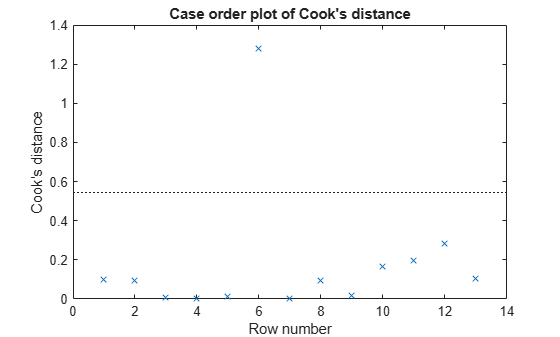# Nonlinear Regression Workflow

This example shows how to do a typical nonlinear regression workflow: import data, fit a nonlinear regression, test its quality, modify it to improve the quality, and make predictions based on the model.

### Step 1. Prepare the data.

Load the `reaction` data.

`load reaction`

Examine the data in the workspace. `reactants` is a matrix with 13 rows and 3 columns. Each row corresponds to one observation, and each column corresponds to one variable. The variable names are in `xn`:

`xn`
```xn = 3x10 char array 'Hydrogen ' 'n-Pentane ' 'Isopentane' ```

Similarly, `rate` is a vector of 13 responses, with the variable name in `yn`:

`yn`
```yn = 'Reaction Rate' ```

The `hougen.m` file contains a nonlinear model of reaction rate as a function of the three predictor variables. For a 5-D vector $b$ and 3-D vector $x$,

`$hougen\left(b,x\right)=\frac{b\left(1\right)x\left(2\right)-x\left(3\right)/b\left(5\right)}{1+b\left(2\right)x\left(1\right)+b\left(3\right)x\left(2\right)+b\left(4\right)x\left(3\right)}$`

As a start point for the solution, take `b` as a vector of ones.

`beta0 = ones(5,1);`

### Step 2. Fit a nonlinear model to the data.

```mdl = fitnlm(reactants,... rate,@hougen,beta0)```
```mdl = Nonlinear regression model: y ~ hougen(b,X) Estimated Coefficients: Estimate SE tStat pValue ________ ________ ______ _______ b1 1.2526 0.86702 1.4447 0.18654 b2 0.062776 0.043562 1.4411 0.18753 b3 0.040048 0.030885 1.2967 0.23089 b4 0.11242 0.075158 1.4957 0.17309 b5 1.1914 0.83671 1.4239 0.1923 Number of observations: 13, Error degrees of freedom: 8 Root Mean Squared Error: 0.193 R-Squared: 0.999, Adjusted R-Squared 0.998 F-statistic vs. zero model: 3.91e+03, p-value = 2.54e-13 ```

### Step 3. Examine the quality of the model.

The root mean squared error is fairly low compared to the range of observed values.

`[mdl.RMSE min(rate) max(rate)]`
```ans = 1×3 0.1933 0.0200 14.3900 ```

Examine a residuals plot.

`plotResiduals(mdl)`The model seems adequate for the data.

Examine a diagnostic plot to look for outliers.

`plotDiagnostics(mdl,'cookd')`Observation `6` seems out of line.

### Step 4. Remove the outlier.

Remove the outlier from the fit using the `Exclude` name-value pair.

```mdl1 = fitnlm(reactants,... rate,@hougen,ones(5,1),'Exclude',6)```
```mdl1 = Nonlinear regression model: y ~ hougen(b,X) Estimated Coefficients: Estimate SE tStat pValue ________ ________ ______ _______ b1 0.619 0.4552 1.3598 0.21605 b2 0.030377 0.023061 1.3172 0.22924 b3 0.018927 0.01574 1.2024 0.26828 b4 0.053411 0.041084 1.3 0.23476 b5 2.4125 1.7903 1.3475 0.2198 Number of observations: 12, Error degrees of freedom: 7 Root Mean Squared Error: 0.198 R-Squared: 0.999, Adjusted R-Squared 0.998 F-statistic vs. zero model: 2.67e+03, p-value = 2.54e-11 ```

The model coefficients changed quite a bit from those in `mdl`.

### Step 5. Examine slice plots of both models.

To see the effect of each predictor on the response, make a slice plot using `plotSlice(mdl)`.

`plotSlice(mdl) ``plotSlice(mdl1) `The plots look very similar, with slightly wider confidence bounds for `mdl1`. This difference is understandable, since there is one less data point in the fit, representing over 7% fewer observations.

### Step 6. Predict for new data.

Create some new data and predict the response from both models.

```Xnew = [200,200,200;100,200,100;500,50,5]; [ypred yci] = predict(mdl,Xnew)```
```ypred = 3×1 1.8762 6.2793 1.6718 ```
```yci = 3×2 1.6283 2.1242 5.9789 6.5797 1.5589 1.7846 ```
`[ypred1 yci1] = predict(mdl1,Xnew)`
```ypred1 = 3×1 1.8984 6.2555 1.6594 ```
```yci1 = 3×2 1.6260 2.1708 5.9323 6.5787 1.5345 1.7843 ```

Even though the model coefficients are dissimilar, the predictions are nearly identical.